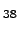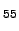Next: CONCLUSIONS Up: ENSEMBLES OF REARRANGEMENT PATHWAYS Previous: Internal Diffusion in LJ   Contents

# Summary

The most important result of this chapter is probably the graph transformation (GT) method. The method works with a digraph representation of a Markov chain and can be used to calculate the first moment of a distribution of the first-passage times, as well as the total transition probabilities for an arbitrary digraph with sets of sources and sinks that can overlap. The calculation is performed in a non-iterative and non-stochastic manner, and the number of operations is independent of the simulation temperature.

We have presented three implementations of the GT algorithm: sparse-optimised (SGT), dense-optimised (DGT), and hybrid (SDGT), which is a combination of the first two. The SGT method uses a Fibonacci heap min-priority queue to determine the order in which the intermediate nodes are detached to achieve slower growth of the graph density and, consequently, better performance. SDGT is identical to DGT if the graph is dense. For sparse graphs SDGT performs better then SGT because it switches to DGT when the density of a graph being transformed approaches the maximum. We find that for SDGT method performs well both for sparse and dense graphs. The worst case asymptotic scaling of SDGT is cubic.

We have also suggested two versions of the SDGT method that can be used in calculations where a greater degree of precision is required. The code that implements SGT, DGT, SDGT, SDGT1 and SDGT2 methods is available for download .

The connection between the DPS and KMC approaches was discussed in terms of digraph representations of Markov chains. We showed that rate constants obtained using the KMC or DPS methods can be computed using graph transformation. We have presented applications to the isomerisation of the LJcluster and the internal diffusion in the LJcluster. Using the GT method we were able to calculate rate constants at lower temperatures than was previously possible, and with less computational expense.

We also obtained analytic expressions for the total transition probabilities for arbitrary digraphs in terms of combinatorial sums over pathway ensembles. It is hoped that these results will help in further theoretical pursuits, e.g. these aimed at obtaining higher moments of the distribution of the first passage times for arbitrary Markov chains.

Finally, we showed that the recrossing contribution to the DPS rate constant of a given discrete pathway can be calculated exactly. We presented a comparison between a sparse-optimised matrix multiplication method and a sparse-optimised version of Algorithm B.1 and showed that it is beneficial to use Algorithm B.1 because it is many orders of magnitude faster, runs in linear time and has constant memory requirements.Next: CONCLUSIONS Up: ENSEMBLES OF REARRANGEMENT PATHWAYS Previous: Internal Diffusion in LJ   Contents
Semen A Trygubenko 2006-04-10